# Legendre transform

The Legendre transform is used to perform a change of variables (see, for example, Ref.  Chapter 4 section 11 Eq. 11.20 - 11.25).

If one has the function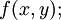$f(x,y);$ one can write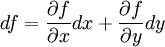$df = \frac{\partial f}{\partial x}dx + \frac{\partial f}{\partial y}dy$

Let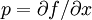$p= \partial f/ \partial x$, and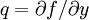$q= \partial f/ \partial y$, thus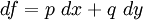$df = p~dx + q~dy$

If one subtracts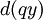$d(qy)$ from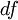$df$, one has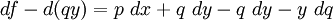$df- d(qy) = p~dx + q~dy -q~dy - y~dq$

or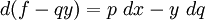$d(f-qy)=p~dx - y~dq$

Defining the function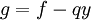$g=f-qy$ then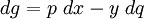$dg = p~dx - y~dq$

The partial derivatives of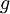$g$ are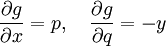$\frac{\partial g}{\partial x}= p, ~~~ \frac{\partial g}{\partial q}= -y$.